Snake Cube
 What is the Snake Cube?  Ways to the Solution The Solution in 17 Steps Twelve Cubes Snake Cube on the Internet .
 To the Main Page "Mathematische Basteleien"

What is the Snake Cube?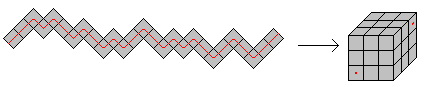The snake cube is a chain of 27 cubes. The cubes are connected by an elastic band running through the middle. There are 17 groups with two or three cubes shown in the drawing.
The aim of the puzzle is to arrange the chain in such a way that there will be a 3x3x3 cube.

Ways to the Solution top
You can only solve the snake cube systematically.First you number the pairs or triplets of cubes from 1 to 17.  You see triplet number 1 on the left. While ordering you should always keep the same orientation in the room.  You fix this orientation by a coordinate system with x-, y- and z-axis.
You learn from a diagram how to arrange the 16 following groups. There are also dead ends with STOP  to show the structure of this puzzle. I will explain the names of the moves by an example. "10-x" means: Arrange the 10th group in direction of
the -x-axis.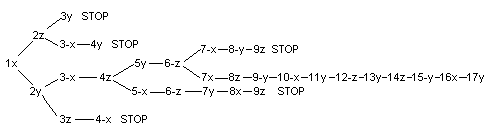If you see the simple diagram you may wonder why this puzzle is so difficult. The reason is that you often have to go back to the last branch. It is difficult to find this place.

There is another solution. You start at the end, but then you will have technical problems. The middle vertical triplet is late and can be laid properly only with difficulties.
There are two more solutions because of symmetry. You can see one of these in a sequence of drawings.

The Solution in 17 Steps   top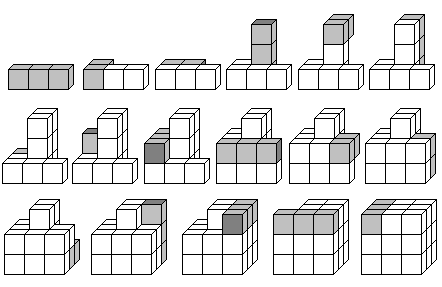........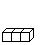........

Twelve Cubes  top
I 've got this nice cube chain. I don't know the name and the manufacturer. Obviously it is similar to the "Kibble Cube".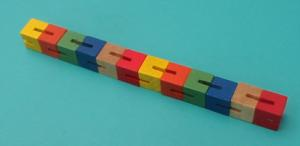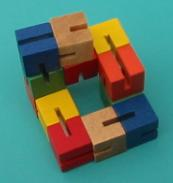...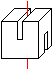... The shape is simple:  There are two slits turned around 90° in opposite faces of one cube. The 12 cubes are connected by  an elastic string along the centre axes. The first and the last cube have a knot inside.
You can give the cube chain any direction in this arrangement.

Chain of 20 cubes
There is a competition for pupils of Primary Schools in Germany called Känguru-Wettbewerb.
A chain of 20 cubes was the prize in 2017.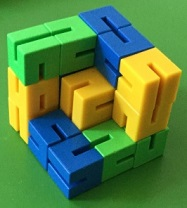Paul built the cube body of 20 cubes.

Chain of 27 cubes
If you would have 27 cubes instead of 12 or 20, you could lay the snake cube above.

Snake Cube on the Internet   top

English

Jaap Scherphuis
Snake CubeKibble Cube

Feedback: Email address on my main page